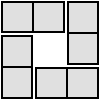#### You may also like### Teddy Town

There are nine teddies in Teddy Town - three red, three blue and three yellow. There are also nine houses, three of each colour. Can you put them on the map of Teddy Town according to the rules?### 4 Dom

Use these four dominoes to make a square that has the same number of dots on each side.### Just Rolling Round

P is a point on the circumference of a circle radius r which rolls, without slipping, inside a circle of radius 2r. What is the locus of P?

# Coordinates of Corners

##### Age 11 to 16

Ahmed found some collections of which made squares.
1. (8,3), (7,8), (2,7), (3,2)
2. (16,19), (18,22), (21,20), (19,17)
3. (4,20), (21,19), (20,2), (3,3)
He noticed that the if you take the second point away from the first point you get the same as if you take the coordinates of the thrid point away from the coordinates of the fourth point. In his first example:
1. $\pmatrix{7\cr 8}-\pmatrix{8\cr 3}=\pmatrix{-1\cr 5}=\pmatrix{2\cr 7}-\pmatrix{3\cr 2}$

Beth from Oxford found a more complete pattern

In order to be a square it needs two things
1. All the sides are the same length
2. All the angles are right angles
These don't depend on where the square is, just where the coordinates are relative to eachother.

If you take the second coordinate away from the first coordinate, the third coordinate away from the second coordinate, the fourth coordinate away from the third coordinate and the first coordinate away from the fourth coordinate, you always get a pattern which looks like
$$\pmatrix{x\cr y},\quad\pmatrix{y\cr-x},\quad\pmatrix{-x\cr -y},\pmatrix{-y\cr x}$$

Why do you think this is? You might want to look at vectors round a square next.

Susie suggested a different way of deciding whether or not the pattern is a square.

If we consider the six distances between the four points and we have four of them of size $a$, for some $a$, and two of them of size $a\times \sqrt{2}$, then we have a square. So we need to compute all the distances between the four points. If they have coordinates $(x_1,y_1), (x_2,y_2), (x_3, y_3), (x_4, y_4)$, then the six distances are $\sqrt{(x_i-x_j)^2 +(y_i-y_j)^2}$ for $1\leq i<j \leq 4$. After that, we arrange them in order and we see whether or not the first four distances are the same, say $a$, and the next two are each of length $a\times \sqrt2$. If they are, then they form a square, otherwise, they don't.

Well done to everyone who sent in a solution. Keep exploring!